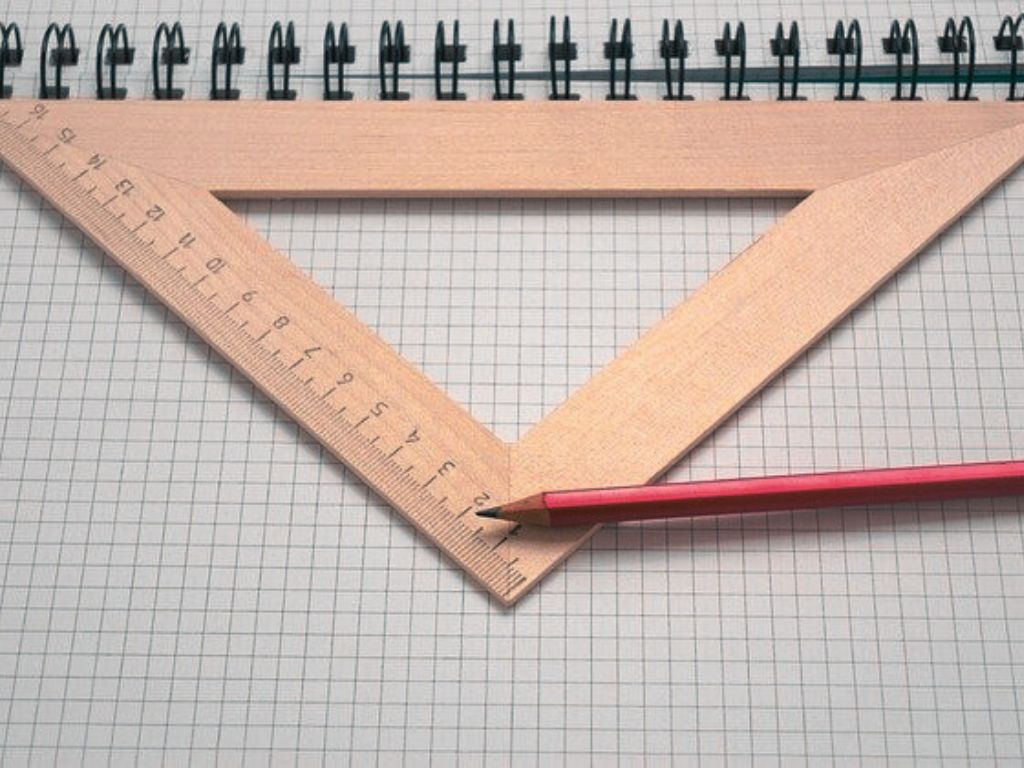## Heron’s Formula Class 9th

Introduction In the previous class, we studied many different types of shapes such as triangles, squares, rectangles, quadrilaterals, etc., and formulae to find their areas. We have already studied how to find the area of a triangle if its base and height are given. We use the formula written below to find the area. Area …